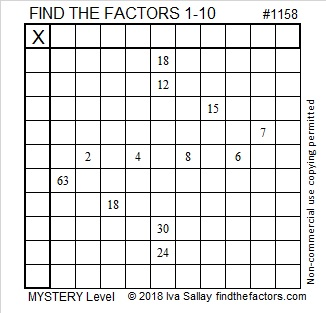# 1158 Mystery Level

The twelve clues in this puzzle are easy to see, but solving the mystery of the puzzle might not be so easy. The clues work together to point to particular factors. Can you figure out where they all go?Print the puzzles or type the solution in this excel file: 10-factors-1148-1160

Now I’ll write a little bit about the number 1158:

• 1158 is a composite number.
• Prime factorization: 1158 = 2 × 3 × 193
• The exponents in the prime factorization are 1, 1, and 1. Adding one to each and multiplying we get (1 + 1)(1 + 1)(1 + 1) = 2 × 2 × 2 = 8. Therefore 1158 has exactly 8 factors.
• Factors of 1158: 1, 2, 3, 6, 193, 386, 579, 1158
• Factor pairs: 1158 = 1 × 1158, 2 × 579, 3 × 386, or 6 × 193
• 1158 has no square factors that allow its square root to be simplified. √1158 ≈ 34.02941158 is the hypotenuse of a Pythagorean triple:
570-1008-1158 which is 6 times (95-168-193)

1158 is 123 in BASE 33 because 33² + 2(33) + 3(1) = 1158

This site uses Akismet to reduce spam. Learn how your comment data is processed.